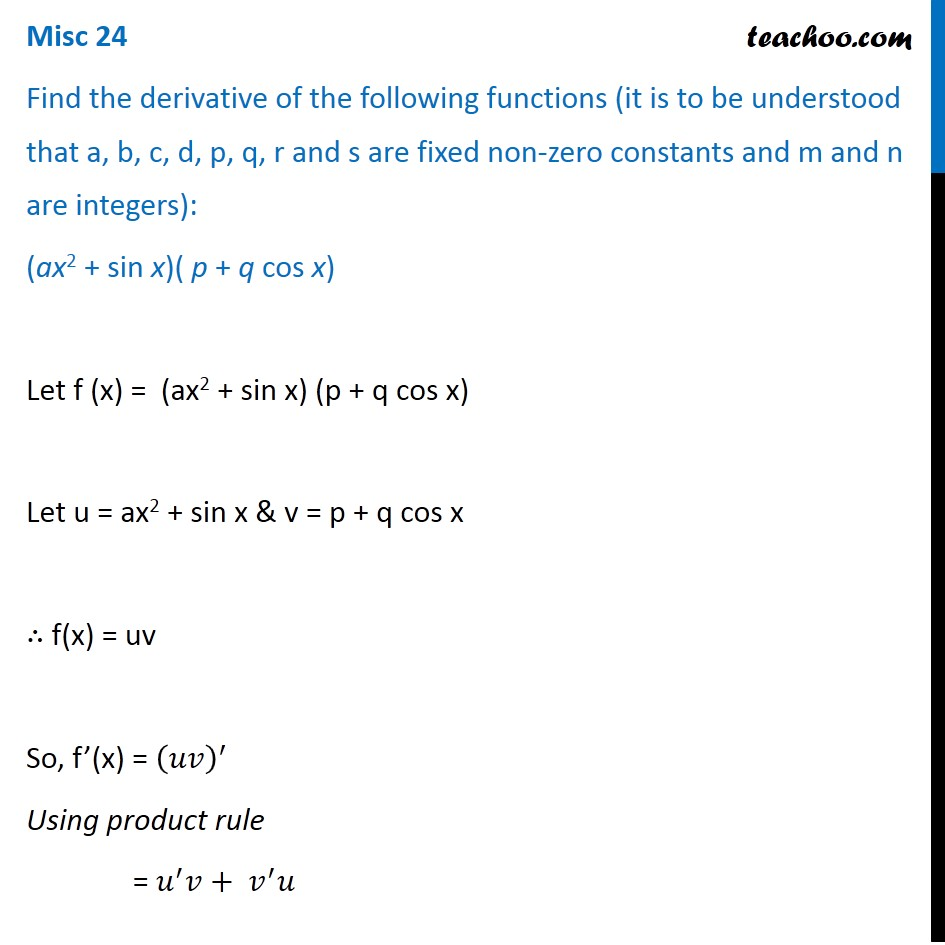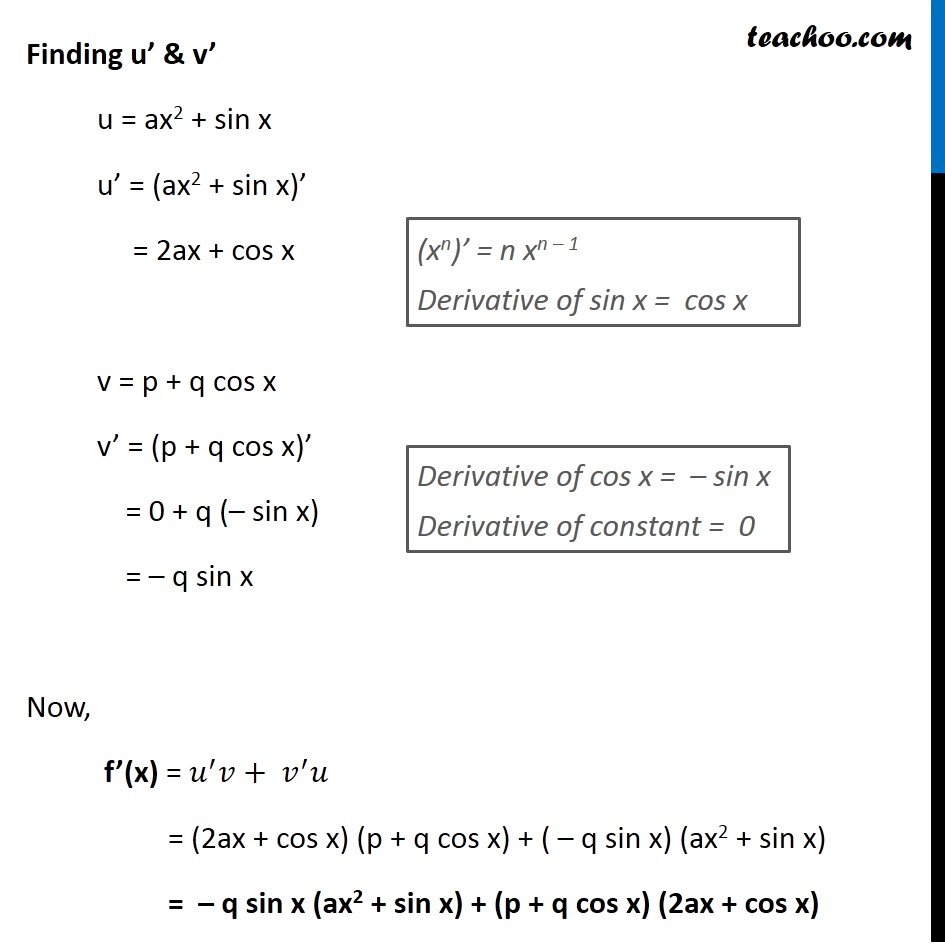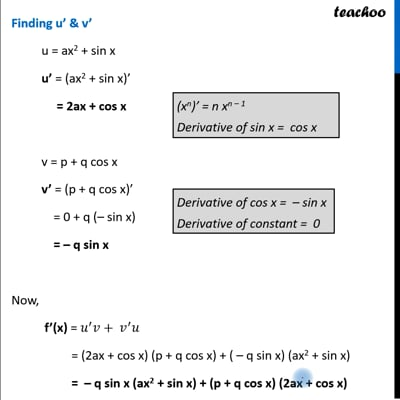Derivatives by formula - sin & cos

Chapter 12 Class 11 Limits and Derivatives
Concept wiseThis video is only available for Teachoo black users

Learn in your speed, with individual attention - Teachoo Maths 1-on-1 Class

### Transcript

Misc 24 Find the derivative of the following functions (it is to be understood that a, b, c, d, p, q, r and s are fixed non-zero constants and m and n are integers): (ax2 + sin x)( p + q cos x) Let f (x) = (ax2 + sin x) (p + q cos x) Let u = ax2 + sin x & v = p + q cos x ∴ f(x) = uv So, f’(x) = (𝑢𝑣)^′ Using product rule = 𝑢^′ 𝑣+〖 𝑣〗^′ 𝑢 Finding u’ & v’ u = ax2 + sin x u’ = (ax2 + sin x)’ = 2ax + cos x v = p + q cos x v’ = (p + q cos x)’ = 0 + q (– sin x) = – q sin x Now, f’(x) = 𝑢^′ 𝑣+〖 𝑣〗^′ 𝑢 = (2ax + cos x) (p + q cos x) + ( – q sin x) (ax2 + sin x) = – q sin x (ax2 + sin x) + (p + q cos x) (2ax + cos x) (xn)’ = n xn – 1 Derivative of sin x = cos x Derivative of cos x = – sin x Derivative of constant = 0